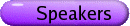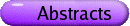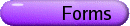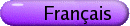Functional Equations and Their Applications
Org: Janos Aczel and Che-Tat Ng (Waterloo)
[PDF]

JOHN BAKER, University of Waterloo, 200 University Avenue W., Waterloo, Ontario N2L 3G1
The Stability of a General Functional Equation
[PDF]

Suppose that V is a vector space over Q, R or C, the scalars a0,b0,...,am,bm are such that ajbk - akbj ¹ 0 whenever 0 £ j < k £ m, B is a Banach space, fk : V ® B for 0 £ k £ m, d ³ 0 and

 || må k=0 fk(ak x+bk y) || £ d    for all x,y Î V.
Then, for each k = 0,1,...,m there exists ck Î B and a "generalized" polynomial function pk : V® B of "degree" at most m-1, such that
 ||fk(x)-ck-pk(x)|| £ 2m+1 d    for all x Î V
and
 må k=0 pk (ak x+bk y) = 0     for all x,y Î V.

Moreover, if V = Rn, B = R or C and, for some j, fj is bounded on a set of positive Lebesgue measure, then every pk is a genuine polynomial function.

WALTER BENZ, University of Hamburg, Department of Mathematics, Bundesstrasse 55, D-20146 Hamburg, Germany
Hyperbolic geometry via functional equations
[PDF]

Let X be a real inner product space of (finite or infinite) dimension at least 2. With implicit notions

(i) of translations t (of X along a fixed axis), and
(ii) of distances d satisfying essentially the functional equations of translation, invariance and additivity,
exactly two geometries with translations t and distances d are characterized up to isomorphism, namely euclidean and Bolyai-Lobachevski geometry over X of dimension dimX.

The methods of the longer proof depend heavily on the theory of functional equations, especially on results of J. Aczél and Z. Daróczy. Our theory in question is part of a book "Geometry of real inner product spaces", forthcoming.

TOM DAVISON, Department of Mathematics, McMaster University, Hamilton, Ontario
D'Alembert's Equation and the Binary Groups
[PDF]

D'Alembert's equation f(xy) + f(xy-1) = 2f(x)f(y) is solved over all finite groups. We introduce the notion of a basic D'Alembert function: one for which f(xy) = f(x) for all x implies that y=1. It is shown that every D'Alembert function factors through a basic D'Alembert function. Then we show that the only finite groups that support a basic D'Alembert function are the cyclic groups (the classical case) and the binary groups:

 á2,m,n ñ: = áR,S,T : R2 = Sm = Tn = RST ñ
in Coxeter's notation. Conversely each of these groups supports a non-classical D'Alembert function.

JEAN-CLAUDE FALMAGNE, University of California, Irvine
Functional Equations and Invariance in Scientific Laws
[PDF]

Two fundamental invariance principles are formulated which enable the derivation of some common physical laws via functional equation techniques. The first invariance principle, called `meaningfulness', is germane to the common practice requiring that the form of a scientific law must not be altered by a change of the units of the measurement scales. The second principle requires that the output variable of the law be `order-invariant' with respect to any monotonic transformation (of one of the input variables) belonging to a particular class of such transformations which is characteristic of the law. These two principles are formulated as axioms of the scientific theory. Three applications are mentioned, which involve: the Lorentz-Fitzerald Contraction, Beer's Law, and the Monomial Laws. The first one is described in some details. If all scientific laws should arguably be meaningful, not all of them are order-invariant in the sense of this work. An example is van der Waals' Equation. Open problems are proposed.

PAL FISCHER, University of Guelph, Guelph, Ontario N1G 2W1
On Schroder equations, linearizability and composition square roots
[PDF]

Results about linearizability and orientation-reversing composition square roots are presented. In addition, a sequence {fn} of strictly increasing and differentiable functions are constructed, defined on an interval I of reals, containing 0 as an interior point with the following properties:

(i) fn(0)=0, fn¢(0)=l, where 0 < l < 1;
(ii) there is no solution of the Schröder equation
 F(fn(x))=lF(x)
on I such that F(0)=0 and F¢(0)=1 for any n positive integer;
(iii) the sequence {fn} converges uniformly on I to lx.

KONRAD HEUVERS, Michigan Technological University, 1400 Townsend Dr., Houghton, MI  49931-1295, USA
A third logarithmic functional equation and Pexider generalizations (joint work with Palaniappan Kannappan)
[PDF]

Let f : ] 0,¥[ ® R be a real valued function on the set of positive reals. Then the functional equations:

 f(x+y) - f(xy) = f(1/x+ 1/y)
 f(x+y) - f(x) - f(x) = f(1/x +1/y)
and
 f(xy) = f(x) + f(y)
are equivalent to each other.

If f,g,h : ] 0,¥[ ® R are real valued functions on the set of positive reals then

 f(x+y) - g(xy) = h(1/x +1/y)
is the Pexider generalization of
 f(x+y) - f(xy) = f(1/x + 1/y).
We find the general solution to this Pexider equation.

If f,g,h,k : ] 0,¥[ ® R are real valued functions on the set of positive reals then

 f(x+y) - g(x) - h(y) = k(1/x +1/y)
is the Pexider generalization of
 f(x+y) - f(x) - f(y) = f(1/x + 1/y).
We find the twice differentiable solution to this Pexider equation.

CHE-TAT NG, University of Waterloo, Waterloo, Ontario N2L 3G1
A functional equation arising from the utility of gambling
[PDF]

In joint work with D. Luce and A. A. J. Marley, on the utility of gambling, an attempt was made to extend the approach of J. R. Meginniss. A functional equation we came across is

 f(a(r)x) + f(a(1-r)x) = xm(r)+f(x)     (r,x Î [0,1])
for some function m : [0,1] ® R. Here a is a homeomorphism on [0,1] and we seek its continuous solution f. We show the application of a uniqueness theorem with which the equation is solved.

PROBLEMS & REMARKS

THOMAS RIEDEL, University of Louisville
Functional equations and an inequality on D+
[PDF]

Let D+ be the space of functions f : [0,¥]® [0,1] such that f(0)=0,f(¥)=1, and f is monotone and left-continuous on (0,¥); this is the space of distance distribution functions. D+ is a complete, completely distributive lattice and hence, continuous lattice. We present our earlier results for certain functional equations on this space and present methods that have been used to solve such equations. We then introduce the notion of a strict inequality defined via the way-below relation from the theory of continuous lattices, and investigate the properties of certain functions on D+ in relation to this inequality. It turns out that some of the methods for solving functional equations can be applied to this question as well.

DILIAN YANG, University of Waterloo
D'Alembert's functional equation on groups
[PDF]

D'Alembert's functional equation is studied in detail and completely solved on compact connected groups. Based on the structure theorem of compact connected groups G, we prove that if G has no any direct factors isomorphic to SU(2) then d'Alembert's equation has only classical solutions; otherwise, non-classical solutions exist and can be factored through one of those direct factors. Our main tools are from representation theory.